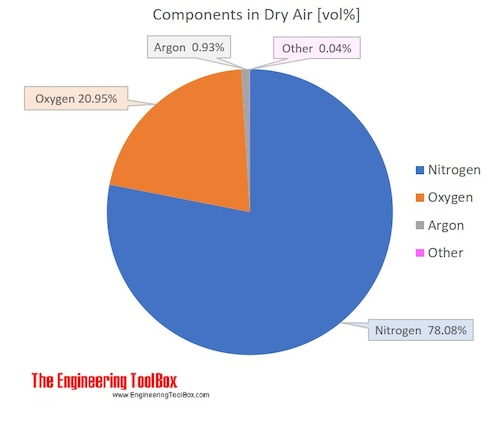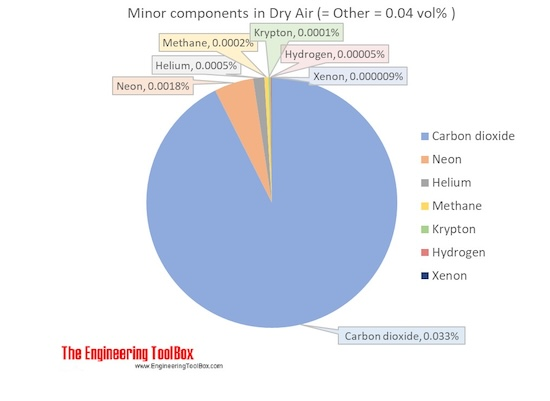Engineering ToolBox - Resources, Tools and Basic Information for Engineering and Design of Technical Applications!

# Air - Molecular Weight and Composition

## Dry air is a mixture of gases where the average molecular weight (or molar mass) can be calculated by adding the weight of each component.

The molecular weight (or molar mass) of a substance is the mass of one mole of the substance, and can be calculated by summarizing the molar masses of all the atoms in the molecule.

### Components in Dry Air

Air is a mixture of several gases, where the two most dominant components in dry air are 21 vol% oxygen and 78 vol% nitrogen. Oxygen has a molar mass of 15.9994 g/mol and nitrogen has a molar mass of 14.0067 g/mol. Since both of these elements are diatomic in air - O2 and N2, the molar mass of oxygen gas is aprox. 32 g/mol and the molar mass of nitrogen gas is aprox. 28 g/mol.

The average molar mass is equal to the sum of the mole fractions of each gas multiplied by the molar mass of that particular gas:

Mmixture = (x1*M1 + ......+ xn*Mn)                                        (1)

where

xi = mole fractions of each gas
Mi = the molar mass of each gas

The molar mass of dry air with oxygen, nitrogen and the other components as indicated below is 28.9647 g/mol.  Composition and content of each gas in air is given in the figures and the table below.For full table with Molar mass an Molar mass in Air - rotate the screen!

 Components in dry air Volume ratio = Molar ratio,  compared to dry air Molar Mass Molar mass in air Name Formula [mol/molair] [vol %] [g/mol][kg/kmol] [g/molair][kg/kmolair] [wt %] Nitrogen N2 0.78084 78.084 28.013 21.873983 75.52 Oxygen O2 0.20946 20.946 31.999 6.702469 23.14 Argon Ar 0.00934 0.934 39.948 0.373114 1.29 Carbon dioxide CO2 0.00033 0.033 44.010 0.014677 0.051 Neon Ne 0.00001818 0.001818 20.180 0.000367 0.0013 Helium He 0.00000524 0.000524 4.003 0.000021 0.00007 Methane CH4 0.00000179 0.000179 16.042 0.000029 0.00010 Krypton Kr 0.0000010 0.0001 83.798 0.000084 0.00029 Hydrogen H2 0.0000005 0.00005 2.016 0.000001 0.000003 Xenon Xe 0.00000009 0.000009 131.293 0.000012 0.00004 Average molar mass of air 28.9647
• 1 kg = 2.2046 lb

### Air Density

The density of dry air can be calculated with the Ideal Gas Law

ρ = P / (R T)                              (1)

where

P = pressure (Pa)

Rair = 287.05 = individual gas constant [J/kg K]

T = absolute temperature [K]

Example: The density of dry air at atmospheric pressure 101.325 kPa (101325 Pa) and 0 oC (= 273.15 K) can be calculated as

ρ = 101325 [Pa] / (287.05 [J/kg K]*273.15 [K])

= 1.292 [kg/m3]

### Water Vapor

Water vapor is almost always present in air. The content may vary and the maximum amount possible of water vapor in dry air depends on the temperature of the air.

Water vapor - H2O - is composed of one oxygen atom and two hydrogen atoms. Hydrogen is the lightest element with a molar mass of 1 g/mol,  while oxygen has 16 g/mol. Thus, the water vapor atom has an molar mass of 18 g/mol, and water vapor is lighter than O2 with 32 g/mol and N2 with 28 g/mol.

• Note! Water vapor in air will dilute the other gases and reduce the total density of the mixture. Dry air is more dense than humid air!

The vapor in air may saturate to droplets when temperature is decreased or pressure is increased.

Humid air containing water molecules as liquid - droplets - may be more dense than dry air or humid air containing water only as vapor.

## Related Topics

• ### Air Psychrometrics

Moist and humid air - psychrometric charts, Mollier diagrams, air-condition temperatures and absolute and relative humidity and moisture content.
• ### Fluid Mechanics

The study of fluids - liquids and gases. Involving velocity, pressure, density and temperature as functions of space and time.
• ### Gases and Compressed Air

Air, LNG, LPG and other common gas properties, pipeline capacities, sizing of relief valves.
• ### Material Properties

Material properties of gases, fluids and solids - densities, specific heats, viscosities and more.

## Related Documents

• ### Air - Composition and Molecular Weight

Dry air is a mechanical mixture of nitrogen, oxygen, argon and several other gases in minor amounts.
• ### Air - Density vs. Pressure and Temperatures

Air density at pressure ranging 1 to 10 000 bara (14.5 - 145000 psi) and constant selected temperatures.
• ### Air - Density, Specific Weight and Thermal Expansion Coefficient vs. Temperature and Pressure

Online calculator, figures and tables showing density, specific weight and thermal expansion coefficients of air at temperatures ranging -100 to 1600 °C (-140 to 2900 °F) at atmospheric and higher pressure - Imperial and SI Units.
• ### Air - Diffusion Coefficients of Gases in Excess of Air

Diffusion coefficients (D12) for gases in large excess of air at temperatures ranging 0 - 400 °C.
• ### Air - Dynamic and Kinematic Viscosity

Online calculator, figures and tables with dynamic (absolute) and kinematic viscosity for air at temperatures ranging -100 to 1600°C (-150 to 2900°F) and at pressures ranging 1 to 10 000 bara (14.5 - 145000 psia) - SI and Imperial Units.
• ### Air - Prandtl Number

Prandtl number for air vs. temperature and pressure.
• ### Air - Properties at Gas-Liquid Equilibrium Conditions

Properties of air change along the boiling and condensation curves (temperature and pressure between triple point and critical point conditions). An air phase diagram included.
• ### Air - Specific Heat Ratio

Specific Heat Ratio of air at temperatures ranging -40 - 1000oC (-40 - 1500oF) at standard atmospheric pressure - Imperial and SI Units.
• ### Air - Specific Heat vs. Pressure at Constant Temperature

Figures and tables with isobaric (Cp) and isochoric (Cv) specific heat of air at constant temperature and pressure ranging 0.01 to 10000 bara.
• ### Air - Specific Heat vs. Temperature at Constant Pressure

Online calculator with figures and tables showing specific heat (Cp and Cv) of dry air vs. temperature and pressure. SI and imperial units.
• ### Air - Speed of Sound vs. Temperature

Speed of sound in air at standard atmospheric pressure with temperatures ranging -40 to 1000oC (-40 to 1500oF) - Imperial and SI Units.
• ### Air - Thermal Conductivity vs. Temperature and Pressure

Online calculator with figures and tables showing air thermal conductivity vs. temperature and pressure. SI and imperial units.
• ### Air - Thermal Diffusivity vs. Temperature and Pressure

Figures and tables withdry air thermal diffusivity vs. temperarure and pressure. SI and Imperial units.
• ### Air - Thermophysical Properties

Thermal properties of air at different temperatures - density, viscosity, critical temperature and pressure, triple point, enthalpi and entropi, thermal conductivity and diffusivity and more.
• ### Dry Air - Thermodynamic and Physical Properties

Thermodynamic properties of dry air - specific heat, ratio of specific heats, dynamic viscosity, thermal conductivity, Prandtl number, density and kinematic viscosity at temperatures ranging 175 - 1900 K.
• ### Dry Air and Water Vapor - Density and Specific Volume vs. Temperature - Imperial Units

Density and specific volume of dry air and water vapor at temperatures ranging 225 to 900 degF (107 to 482 degC).
• ### Gas Mixtures - Properties

Gas mixtures and the ideal gas law, mass calculations, the individual gas constant and density.
• ### Gases - Densities

Densities and molecular weights of common gases like acetylene, air, methane, nitrogen, oxygen and others.
• ### Gases - Molar Specific Heats

Molar specific heats for some common gases at constant volume.
• ### Humid Air vs. the Ideal Gas Law

Pressure, temperature and volume in a perfect ideal gas like moist air (air with water vapor).
• ### Moist Air - Density vs. Water Content and Temperature

Density of the mix of dry air and water vapor - moist humid air.
• ### Moist Air - Mole Fraction of Water Vapor

Mole fraction of water vapor is the ratio of water molecules to air and water molecules.
• ### Mole and the Avagadro's Number

The mole is the SI base unit for an amount of a substance.
• ### Molecular Weight of Substances

Definition and molecular weight (molar mass) of some common substances.
• ### Nitrogen - Thermophysical Properties

Chemical, Physical and Thermal Properties of Nitrogen - N2.
• ### Non-ideal gas - Van der Waal's Equation and Constants

The van der Waals constants for more than 200 gases used to correct for non-ideal behavior of gases caused by intermolecular forces and the volume occupied by the gas particles.
• ### Oxygen - Thermophysical properties

Chemical, Physical and Thermal Properties of Oxygen - O2.
• ### The Ideal Gas Law

The relationship between volume, pressure, temperature and quantity of a gas, including definition of gas density.
• ### Total and Partial Pressure - Dalton's Law of Partial Pressures

How to calculate total pressure and partial pressures for gas mixtures from Ideal Gas Law.
• ### Universal and Individual Gas Constants

The Universal and Individual Gas Constants in fluid mechanics and thermodynamics. Individual gas constants for the most common gases.

## Engineering ToolBox - SketchUp Extension - Online 3D modeling!

Add standard and customized parametric components - like flange beams, lumbers, piping, stairs and more - to your Sketchup model with the Engineering ToolBox - SketchUp Extension - enabled for use with older versions of the amazing SketchUp Make and the newer "up to date" SketchUp Pro . Add the Engineering ToolBox extension to your SketchUp Make/Pro from the Extension Warehouse !

We don't collect information from our users. More about

## Citation

• The Engineering ToolBox (2004). Air - Molecular Weight and Composition. [online] Available at: https://www.engineeringtoolbox.com/molecular-mass-air-d_679.html [Accessed Day Month Year].

Modify the access date according your visit.

9.19.12